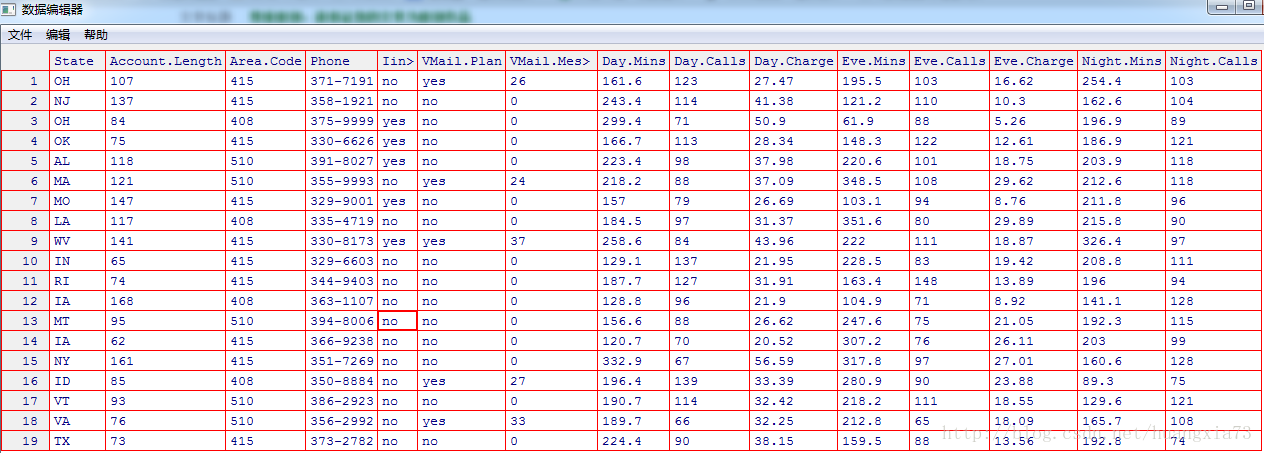# 数据挖掘方法之六：解读逻辑回归

## 一 使用数据

   call_consumer<-read.table(file="d:/LabData/RData/churn.txt",header=TRUE,sep=",")
Warning message:
In read.table(file = "d:/LabData/RData/churn.txt", header = TRUE,  :
incomplete final line found by readTableHeader on 'd:/LabData/RData/churn.txt'
> edit(call_consumer)## 二 .解读逻辑回归模型

• 一个两分预测变量的模型
• 多分预测变量
• 连续的预测变量

### 2.1 两分预测变量模型

语音邮箱=否(x=0) 语音邮箱=是(x=1) 合计

OR=0.095/0.2007=0.47

• 对于一个拥有此套餐的客户，估计他的流失概率为： $\pi(1)=0.0868$（也可以直接计算 P(流失|语音邮箱计划)=80/922=0.0868)，这一概率比数据集中给出的客户流失的总比例14.5%要小，说明开通语音邮箱套餐有利于减少客户流失。

• 对于一个没有拥有此套餐的客户，估计他的流失概率为：$\pi(0)=0.16715$ （也可以直接计算 P(流失\｜语音邮箱计划)=403/2411=0.16715，这一概率比数据集中给出的客户流失的总比例14.5%要高，说明没有开通语音邮箱套餐对于客户流失不大。
• 进一步地，可以利用Wald检验法检验语音邮箱套餐参数的显著性。这里, $b_1$ =-0.747795, $SE(b_1)$ =0.129101得 $Z_{wald}=-0.747795/0.129101=-5.79$ P值为P(|Z|>-5.79)趋近于0

### 2.2 多分预测变量模型

• 0个或1个客户服务电话：CSC＝低
• 2个或3个客户服务电话：CSC＝中
• 4个以上客户服务电话：CSC＝高

CSC-中 csc-高

CSC-低 CSC-中 CSC-高 合计

• 对于CSC－中：$\bar {OR}＝ｅ^{b1}＝ｅ^{-0.03698}=0.96$
• 对于CSC－高：$\bar {OR}＝ｅ^{b2}＝ｅ^{2.11844}=8.32$

• 对于那些很少拨打客服电话的客户：$g(x)=e^{-2.051-0.036989(0)+2.11844(0)}=e^{-2.501}$ 概率为：$\pi(x)=\frac{e^{-2.501}}{1+e^{-2.501}}=0.114$ 。此概率比全部数据样本集中客户流失的概率14.5%要小。这表明这一类客户的流失率一定程度上比总体客　　户的流失率要小。
• 对于拨打客服电话处于中等水平的客户，同上，此时 $g(x)=e^{-2.051-0.036989(1)+2.11844(0)}=e^{-2.088}$ 注意系数的差别,上一条中的系数是0，0，这个是1，0
• 对于经常拨打客服电话的客户，同上，此时 $g(x)=e^{-2.051-0.036989(0)+2.11844(1)}=e^{-2.501}$ 注意系数的差别,上一条中的系数是1，0，这个是0，1</font>

#### Wald检验

• 对于CSC－中 的参数进行Wald检验，$b_1=-0.036989,SE(b_1)=0.11771$ 　故而， $Z_{wald}＝-0.036989/0.117701=-0.31426$

•  对于CSC－高的参数进行Wald检验，$b_1=2.11844,SE(b_1)=0.142380$故而$Z_{wald}=2.11844/0.142380=14.88$此时，P值P( Z >14.88)=0.000，显著，表明CSC－高与CSC－低的差异能有效预测客户流失。

### 2.3　解读连续预测变量模型

• 对于一个日使用分钟数为100的顾客流失的概率估计为：

• 对于一个日使用分钟数为300的顾客流失的概率估计为：

“日使用分钟数”，这一实例的偏差Ｇ为：

## 三.多元逻辑回归

• 针对单个变量的挑选：Wald检验某个变量是否有助于预测
• 针对多个变量总体挑选：总体显著性Ｇ